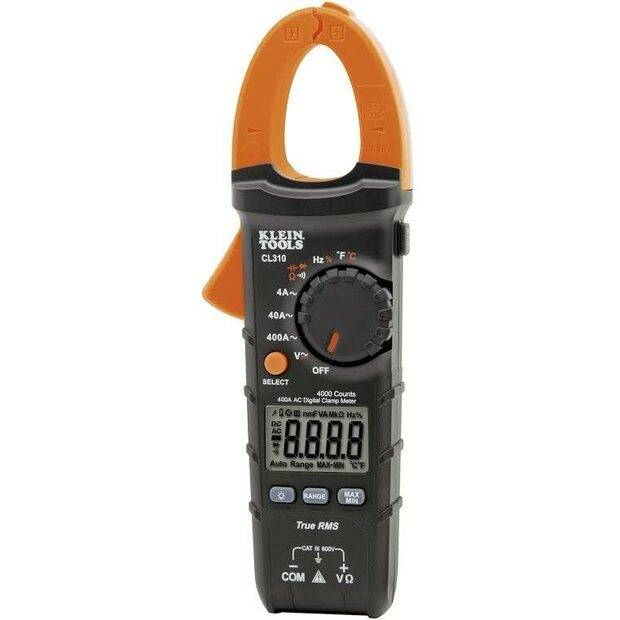# 3 phase wire amperage

• Awwtumn
Think of the shared conductors like this:
-
If you are familiar with an amp clamp like these:Then you realize you can clamp around a wire and measure the current it's carrying. You can also clamp around several wires and measure the current vectorially summed together. So if you had a situation where you had three separate transformer/load pairs all 120° out of phase you could group several wires and find your meter would tell you 17.33 amps when measuring two 10 amp loads from sources 120° out of phase. Maybe this makes more sense for you?

•russ_watters
Do you have any experience with circuit analysis? Kirchhoff's current laws? Stuff like that?
-
With the 10 amp loads in delta you have three 10 amp loads each being driven by three separate transformers. It just so happens that those three loads and sources are sharing feeder conductors. If you understand the vectorial relationships of the three sources and understand basic circuit analysis you will understand why the currents come out the way they do in the feeder conductors.
-
In your case of three 10 amp loads in series, what you really have is a single 10 amp load which is driven by a single transformer. There is the other transformer connected, but only one lead of the second transformer. The second transformer is contributing nothing in this case. Knowledge of basic circuit analysis would tell you this. So you have have a 10 amp load at whatever voltage is driving it, that's it.

For the three 10A loads in delta. How do you compute for the total power drawn in say the 3 delta transformers source? I just want to know how it compares to using just one transformer with three 10A loads in series. Is it more or less?

In commercial building 3-phase electric metering, they use Demand charge where the rate depends on the highest or peak kW used. So let's say all the one phase aircons were concentrated in one transformer or phase of the 3. Won't this increase the demand charge by increasing the peak kW used and hence the bill? Or does it somehow average out in all the 3 phase so it doesn't matter? This is what I want to know. Thanks.

Last edited:
For the three 10A loads in delta. How do you compute for the total power drawn in say the 3 delta transformers source? I just want to know how it compares to using just one transformer with three 10A loads in series. Is it more or less?

In commercial building 3-phase electric metering, they use Demand charge where the rate depends on the highest or peak kW used. So let's say all the one phase aircons were concentrated in one transformer or phase of the 3. Won't this increase the demand charge by increasing the peak kW used and hence the bill? Or does it somehow average out in all the 3 phase so it doesn't matter? This is what I want to know. Thanks.
Three 10 amp loads in delta are simply three 10 amp loads driven by their source voltage 240 V * 10 A = 2400 watts. Three separate loads so a total load of 7200 watts that the Poco will bill you for.
-
Your question about three 10 amp loads in series makes no sense as I have already told you. The lights, motors, etc. used in mains wiring are not in series. If you have three 10 amp loads that are NOT balancing the delta supply there will either be all loads on one phase or two on one phase and one on another phase. Each load will have 240 volts across it and another total power for all three combined will still be 7200 watts.

@Awwtumn do you understand your electric bill? How many meters are listed?